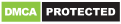# JavaScript ES6 Three Dots Syntax (...): Spread vs. Rest Operators

ES6 has introduced the three dots (`...`) syntax in JavaScript and Node which can be used, depending on the context, as a Spread oprator or a Rest operator. Let's see the difference between these two operators in more details.

In a previous post, we've seen what's the Spread operator and that we can use it to expand or unpack the elements of a JavaScript iterable in an appropriate place like inside another array or object literal.

The Rest operator uses the same syntax but does the opposite of the Spread operator. It reduces or collects values into an array.

The best way to grasp the difference between the two operators is through some examples.

## The Spread Operator & the `apply()` method

Let's assume, you want to print the elements of the following array in the console:

``````const letters = ['a', 'b', 'c'];
``````

You would call the `console.log.apply()` method as follows:

``````console.log.apply(this,letters);
``````

That's because using the `console.log()` directly will print the array as a single object.

In ES6, the Spread oparator allows you to do the same but in a much readable way:

``````console.log(...letters);
``````

We spread the values of the `letters` array and we print them, just like if we are doing something like:

``````console.log("a", "b", "c");
``````

## Deep Copying an Array with the Spread Operator

We can use the Spread operator to copy an array into another. For example:

``````letters2 = [...letters];
``````

The `letters2` array is a deep copy of the `letters` array which means we can change the `letters2` array without affecting the first array.

## Concatenating Arrays with the Spread Operator

We can use the Spread operator to concatenate two or more arrays into one array. Since the Spread operator unpack an array when it's applied to it we can do something like:

``````const a = [1, 2, 3 ];
const b = [4, 5, 6];

const ab = [...a, ...b];
``````

We simply extract the values of the `a` and `b` arrays into the new `ab` array.

## Using the Spread Operator for Passing function arguments

Let's suppose, you have a function that expects many individual arguments. For example, let's this one:

``````function print(a, b, c){
return console.log(a,b,c);
}
``````

And we have those values that we want to print in some array:

``````const letters = ['a', 'b', 'c'];
``````

Instead of referencing each array member and passing it as a single argument which is quite tedious:

``````print(letters, letters, letters);
``````

We can simply use the Spread operator like the following:

``````print(...letters);
``````

## Transforming a String into an Array with the Spread Operator

The Spread operator works with any iterable such as literal JavaScript objects and also strings. So, we can make use of its magic to quickly transform a string into an array of characters. For example:

``````const str = "my string";

const strarr = [...str];
``````

## The Rest Operator in JavaScript

As we mentionned before, both the Spread and Rest operator uses the three dots syntax prepended at the name of the symbol.

In the previous section, we've seen various examples of the Spread operator which expands iterables into their elements. Now, let's see the Rest operator by example.

Just like its name suggests, the Rest operator collects the rest or the remaining of elements into an array.

### Using Variarble Arguments (Variadic) Functions in JavaScript

Let's suppose, you want to define a function that could take a variable number of arguments. You would use the `arguments` object:

``````function f(){
return arguments;
}

f(1, 'a', 'hello');
``````

The `arguments` object is an array-like object, as such as you can not use Array methods with it. You also can not use with Arrow functions which are now very common between JS developers to define functions.

A better alternative is to use the Rest operator. For example:

``````
function f(...args){
return args;
}
``````

## Conclusion

In this quick post, we've seen the difference between the Spread and Rest operators in JavaScript (ES6) which both makes use of the same three dots syntax. As a recap, you can use the Spread operator if you like to expand (unpack) the elements of an iterable like object, array or string literal. In the contrary, you can use the Rest operator to collect multiple elements into an array.

Note: We also publish our tutorials on Medium and DEV.to. If you prefer reading in these platforms, you can follow us there to get our newest articles.

You can reach the author via Twitter:is a web developer with 5+ years of experience and technical author with an engineering degree on software development. You can hire him with a click on the link above or contact him via his LinkedIn account. He authored technical content for the industry-leading websites such as SitePoint, Smashing, DigitalOcean, RealPython, freeCodeCamp, JScrambler, Pusher, and Auth0. He also co-authored various books about modern web development that you can find from Amazon or LeanpubGet our Learn Angular 8 in 15 Easy Steps ebook in pdf, epub and mobi formats, plus a new Angular 8 tutorial every 3 days.

## Online Tools

SVG URL Encoder Bootstrap Navbar Generator

## Get our books